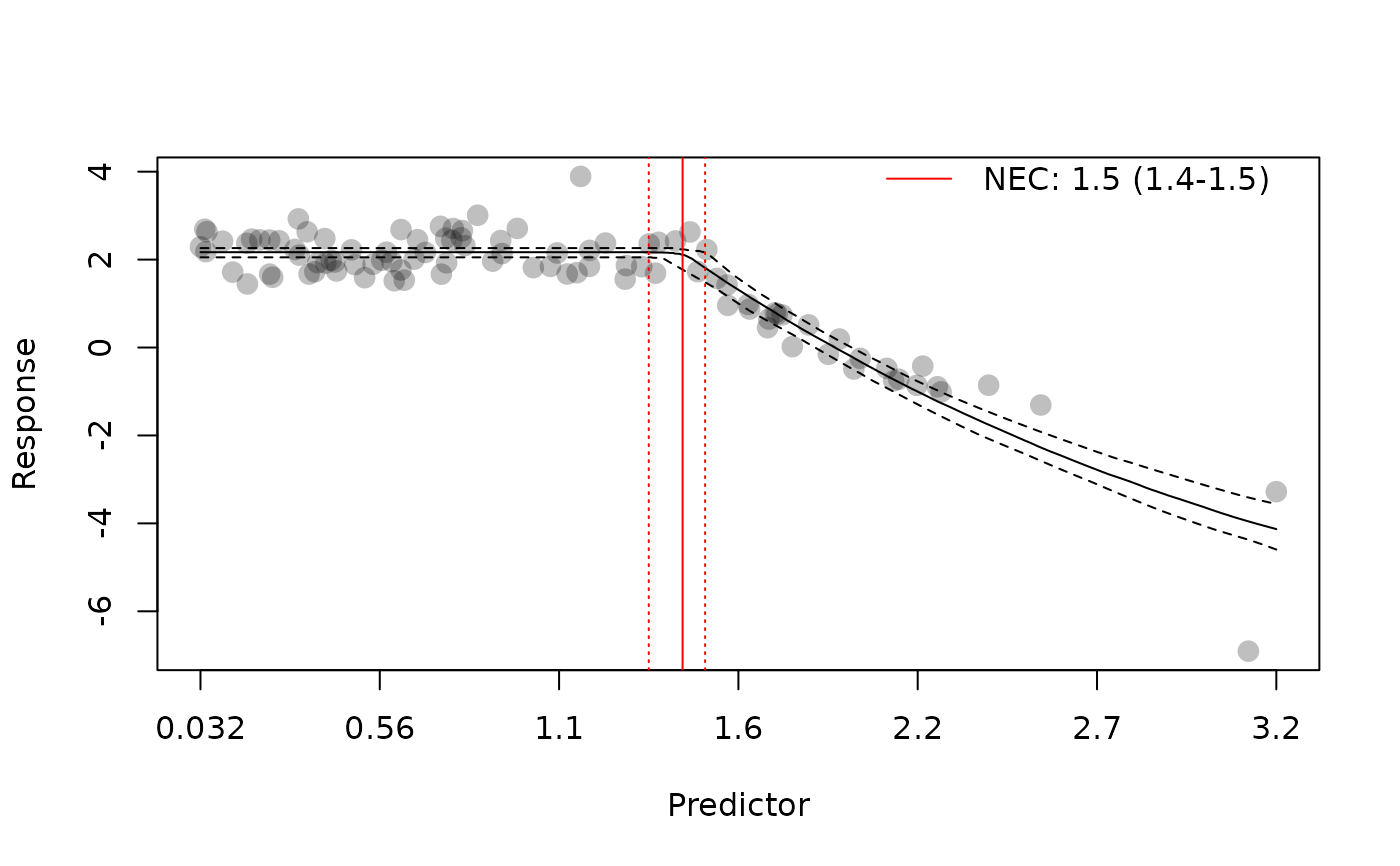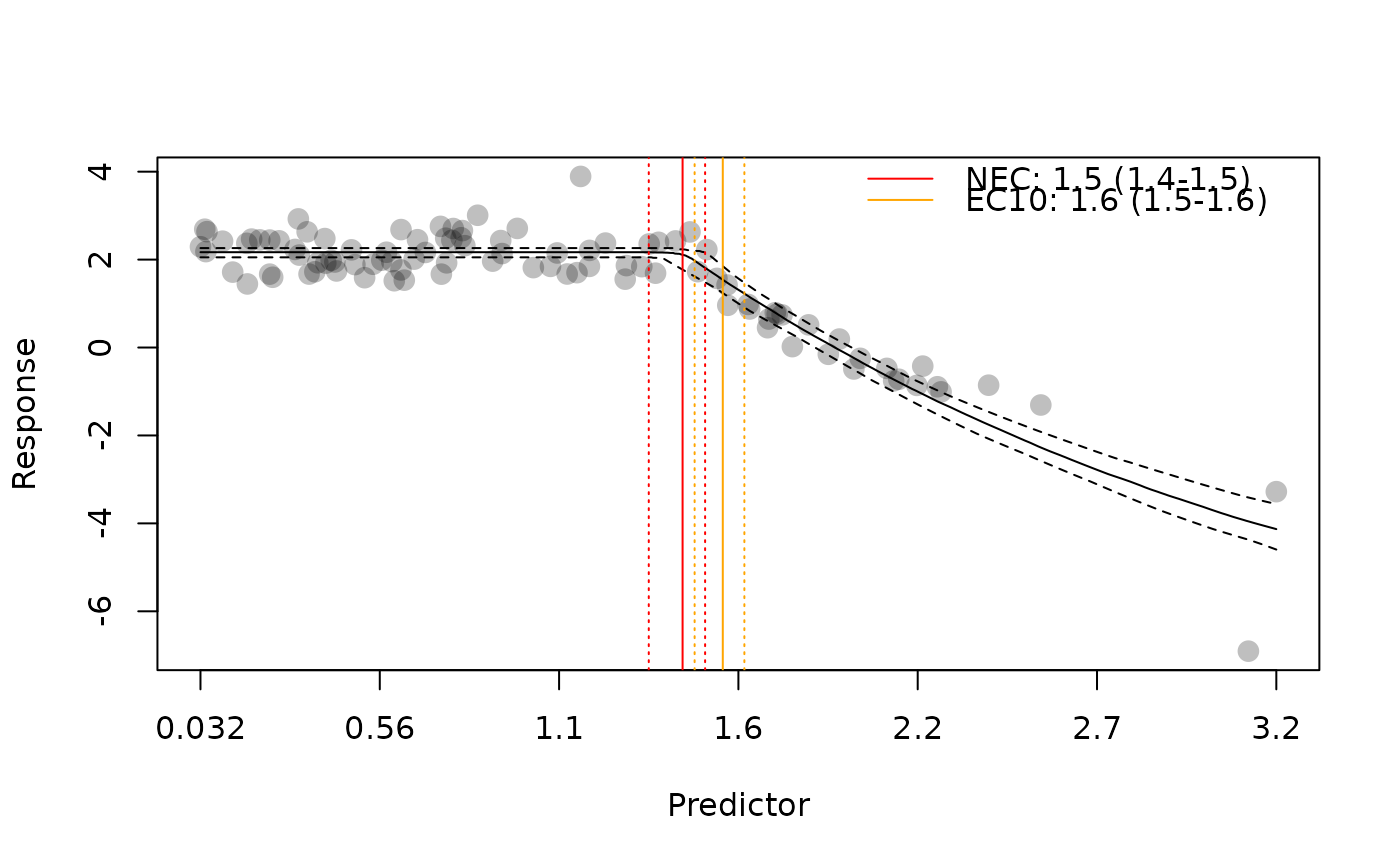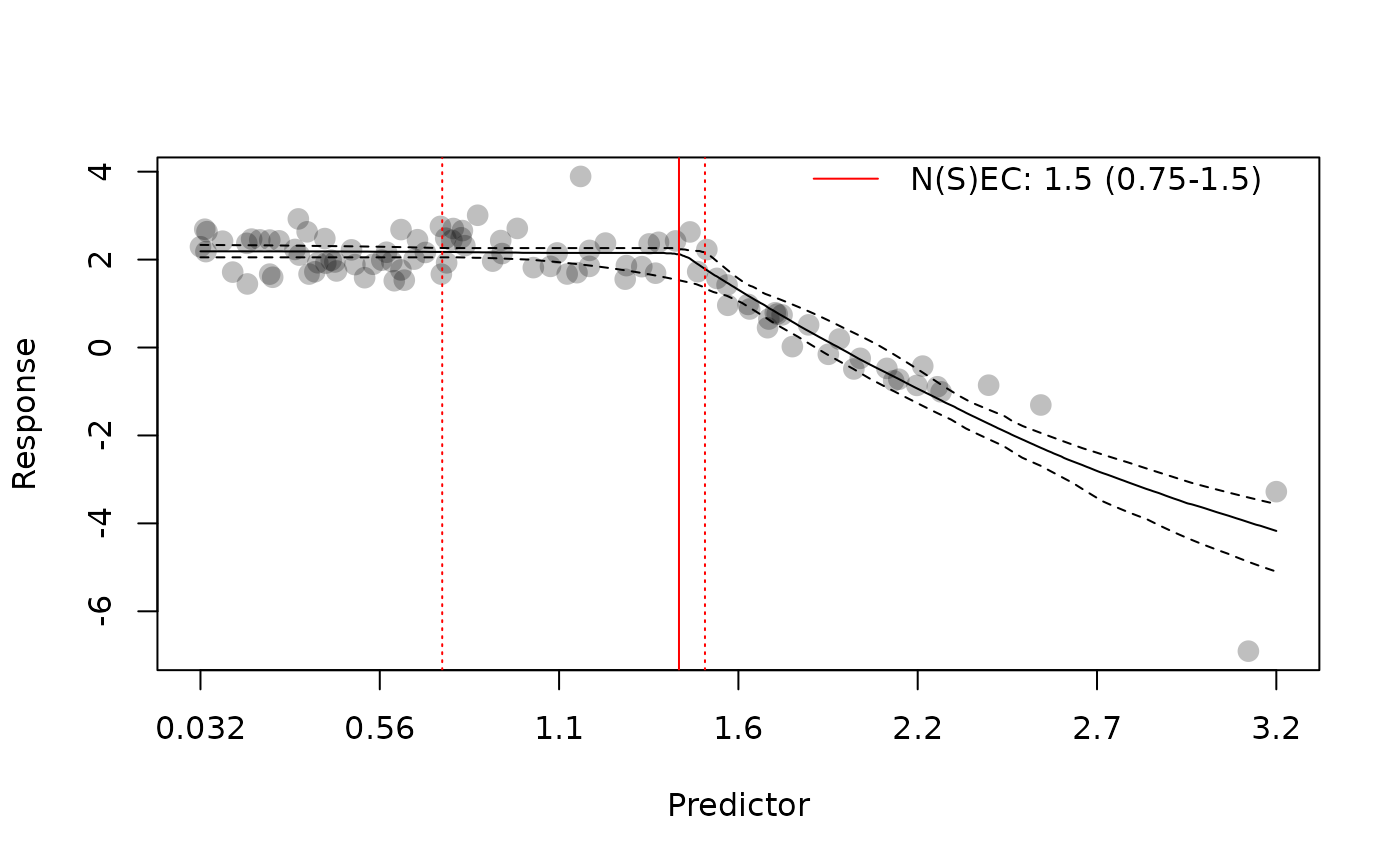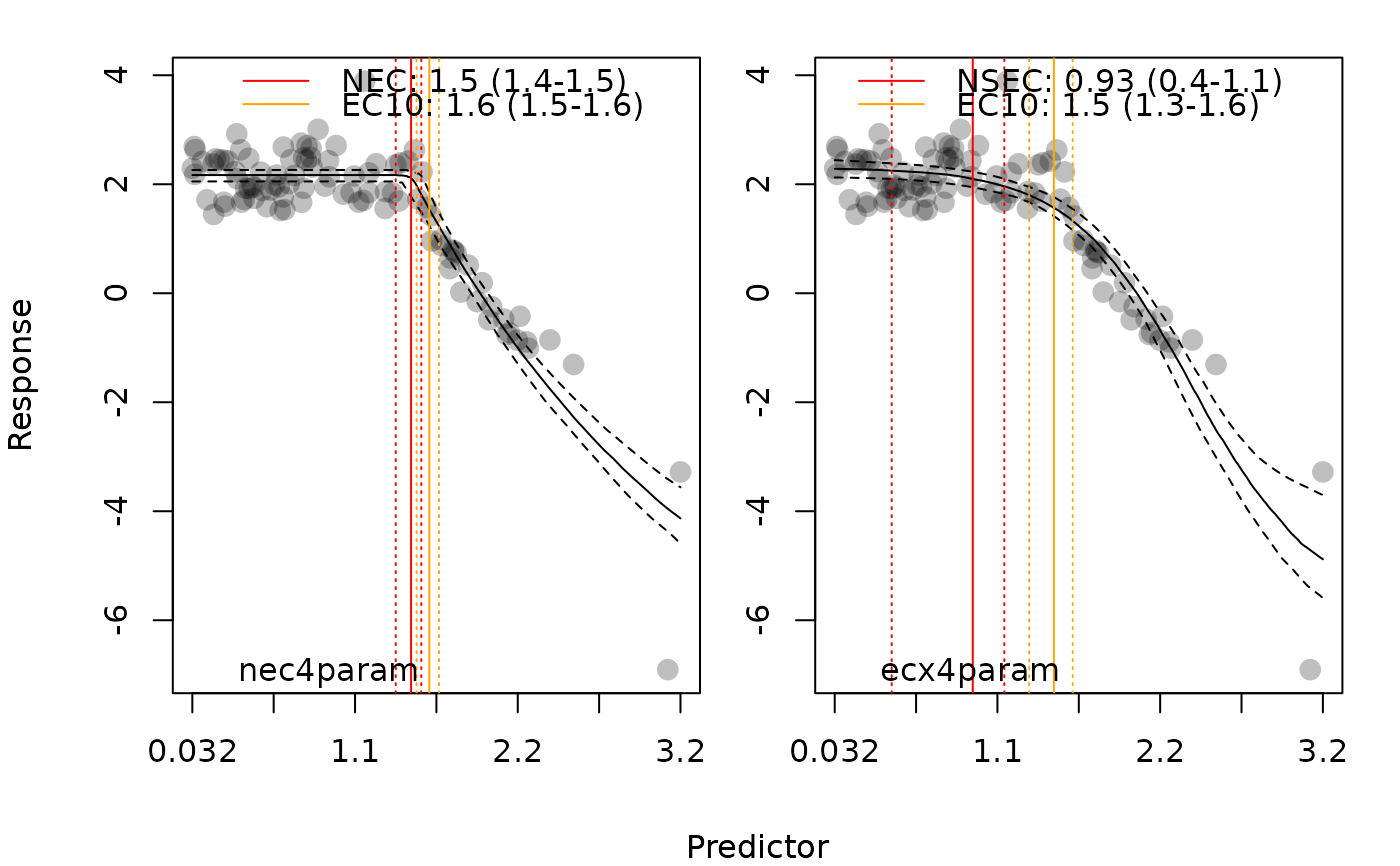Generates a plot for objects fitted by bnec. x should be of class bayesnecfit or bayesmanecfit.

# S3 method for bayesnecfit
plot(
x,
...,
CI = TRUE,
position_legend = "topright",
xform = identity,
lxform = identity,
jitter_x = FALSE,
jitter_y = FALSE,
ylab = "Response",
xlab = "Predictor",
xticks = NA
)

# S3 method for bayesmanecfit
plot(
x,
...,
CI = TRUE,
position_legend = "topright",
xform = identity,
lxform = identity,
jitter_x = FALSE,
jitter_y = FALSE,
ylab = "Response",
xlab = "Predictor",
xticks = NA,
all_models = FALSE
)

## Arguments

x

An object of class bayesnecfit or bayesmanecfit.

...

Additional arguments to plot.

CI

A logical value indicating if credibility intervals on the model fit should be plotted, calculated as the upper and lower bounds of the individual predicted values from all posterior samples.

A logical value indicating if the estimated NEC value and 95% credible intervals should be added to the plot.

position_legend

A numeric vector indicating the location of the NEC or EC10 legend, as per a call to legend.

A logical value indicating if an estimated EC10 value and 95% credible intervals should be added to the plot.

xform

A function to be applied as a transformation of the x data.

lxform

A function to be applied as a transformation only to axis labels and the annotated NEC / EC10 values.

jitter_x

A logical value indicating if the x data points on the plot should be jittered.

jitter_y

A logical value indicating if the y data points on the plot should be jittered.

ylab

A character vector to use for the y-axis label.

xlab

A character vector to use for the x-axis label.

xticks

A numeric vector indicate where to place the tick marks of the x-axis.

all_models

A logical value indicating if all models in the model set should be plotted simultaneously, or if a model average plot should be returned.

## Value

A plot of the fitted model.

## Examples

# \donttest{
library(bayesnec)
nec4param <- pull_out(manec_example, "nec4param")
#> Pulling out model(s): nec4param
# plot single models (bayesnecfit)
plot(nec4param)